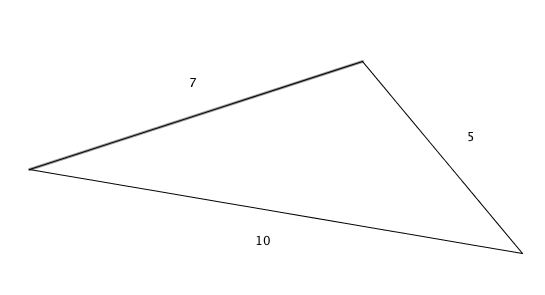Geometry

# Perimeter

Khom drew a triangle has side lengths of 5, 7 and 10. What is the perimeter of this triangle?What is the perimeter of a square with side length 3?

What is the perimeter (in inches) of a rectangle which measures 8 inches by 15 inches?

A regular hexagon has a side of length 7. What is its perimeter?

Scalene triangle $$ABC$$ has side lengths $$AB = 10$$, $$BC = 14$$ and $$CA = 16$$. What is the perimeter of $$ABC$$?

×